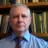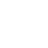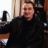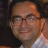## Склад секціїAlexander L. Yampolsky Docent (associate professor) of department of fundamental mathematics, doctor of sciences in physics and mathematics, docent (associate professor)Petro G. Dolya Docent (associate professor) of department of theoretical and applied computer science , phd for industriesGrigoriy C. Kurinniy Docent (associate professor) of department of theoretical and applied computer science , phd in mathematics, docent (associate professor)Dmytro V. Bolotov Doctor of sciences in physics and mathematics, senior researcher of iltpeVasyl O. Gorkavyy Doctor of sciences in physics and mathematics, docent (associate professor)

## Schedule for today

Week schedule# Petro G. Dolya

Docent (associate professor) of department of theoretical and applied computer science , phd for industries

Peter G. Dolya associate professor of mechanical and mathematical department, V.N. Karazin Kharkiv National University, Ukraine.

In 1978 year he completed mechanical and mathematical department of Kharkov State University. He have defended the Ph.D. thesis on applied geometry. He is the author of the monograph which theme is geometrical modeling of the fabric composites on curvilinear surfaces. He is the author of 33 scientific articles.

Peter G. Dolya is a specialist in the field of mathematical modeling and especially in geometrical modeling. The main research interests are piecewise functions and their applications in geometrical modeling, mechanics and mathematical physics. He have long-term experience of creating of application program, add-ons and packages with various programming languages. Besides, he have wide experience of programming on VBA in systems of engineering graphs, such as ACAD, Mechanical Desktop and in Microsoft Office applications.

Length of teaching experience is more than 30 years.

For the students of application department of geometry sub-faculty Peter Dolya lectures some courses. Some of them introduces students to main ability of the modern systems of symbolic mathematics such as MATHEMATICA and MAPLE and acquaint with mathematical system MATLAB. The other courses submit application of this systems in mathematical researches.

• Special course «Analytical methods of geometric simulation» acquaints students with the modern computer methods of modeling of curves, surfaces and other geometrical objects. In it the theory of splines and its application to modeling of curves and surfaces is stated. In the module acquaintance to theory of R-functions and its application to modeling of piecewise smooth continuous curves and surfaces is given. Method of piecewise smooth continuous functions and its application for solution of inverse problems of an analytical geometry are stated.
• Special course «Foundations of mathematical modeling and computing experiment» contains materials for acquaintance of students to the modern methods of computing experiment. With application of the modern systems of computer mathematics mathematical models from various fields are explored. The base software are MATHEMATICA, MAPLE and MATLAB. Main explored models concern to fields of modeling of mechanical equipment (a solution of the differential equations), calculations of elements of constructions (the equation of mathematical physics), calculation of the composite constructions (system of the linear and differential equations) and to geometrical modeling (computer geometry). Peculiarity of application of systems of symbolic mathematics for solution of engineering problems and new possibilities of visualization of the received solutions (animation of graphs, three-dimensional surfaces, animation of moving of elements of constructions, visualization scalar and vector fields, etc.) are considered.The basic ideas of application of the finite-element method and boundary element method are considered.
• Special course «Creating packages and add-ons for systems of engineering graphs, office and mathematical software» based on Peter Dolya programming experience. The course contains subjects that associated with enhancement of interface and computational power of classical software such as Microsoft Word, Microsoft Excel, AutoCAD, Maple, Mathematica. The course contains such sections: application of VBA in Microsoft Office and AutoCAD, modes of development of packages for Maple and Mathematica.
• In the special course «Fundamentals of computing in Mathematica system» subjects of application of the modern system of symbolic mathematics are considered. Such themes are included: symbolic transformations, linear algebra, scientific graph, mathematical analysis, differential equations, fundamentals of programming in system Mathematica. Review of a system of symbolic mathematics Maple is given.

Of late years Peter Dolya prepares and gives two courses which connected with modern areas of applied mathematics. Special course «Mathematical Foundations of Computer Tomography» is given to students of application department of geometry sub-faculty ant to students of specialty of Computer Science. New special course «Mathematical Methods of Image Processing» is given to fourth year students of specialty of Computer Science.